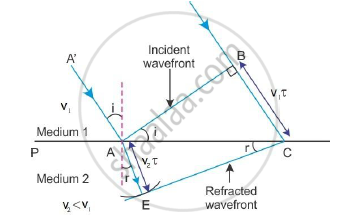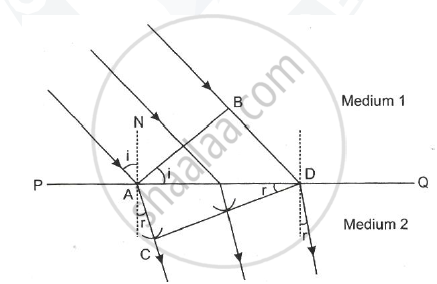# Derive Snell’S Law on the Basis of Huygen’S Wave Theory When Light is Travelling from a Denser to a Rarer Medium. - Physics

Derive Snell’s law on the basis of Huygen’s wave theory when the light is travelling from a denser to a rarer medium.

Using Huygen’s wave theory, derive Snell’s law of refraction

#### Solution 1

Let PP′ represent the surface separating medium 1 and medium 2, as shown in the figure below.Let v1 and v2 represent the speed of light in medium 1 and medium 2, respectively. We assume a plane wavefront AB propagating in the direction A′A incident on the interface at an angle ‘i’ as shown in the figure. Let τ be the time taken by the wavefront to travel the distance BC. Thus, BC = v1t

To determine the shape of the refracted wavefront, we draw a sphere of radius v2τ from the point A in the second medium (the speed of the wave in the second medium is v2 > v1).

Let CE represent a tangent plane drawn from the point C on to the sphere. Then, AE = v2τ and CE would represent the refracted wavefront. If we now consider the triangles ABC and AEC, we readily obtain

sini=(BC)/(AC)=(v_1t)/(AC)

sinr=(AE)/(AC)=(v_2t)/(AC)

where i and r are the angles of incidence and refraction, respectively. Therefore, from equations (1) and (2), we get

sini/sinr=(v_1t)/(AC)xx(AC)/(v_2t)=v_1/v_2

From the above equation, if r > i (i.e. if the ray bends away from the normal), the speed of the light wave in the second medium (v2) will be greater than the speed of the light wave in the first medium (v1).

Now, if c represents the speed of light in vacuum, then

n_1=c/v_1

n_2=c/v_2

These are known as the refractive indices of medium 1 and medium 2, respectively. In terms of the refractive indices, equation 3 can be written as

sini/sinr=v_1/v_2=c/n_1xxn_2/c

∴ n1sini=n2sinr

This is the Snell’s law of refraction.

#### Solution 2

Laws of Refraction: Consider a plane wavefront AB incident on a surface PQ separating two
media (1) and (2). The media (1) is rarer, having refractive index n1, in which the light travels with a velocity c1. The medium (2) is denser, having refractive index n2, in which the light travels with a velocity c2.At time t = 0, the incident wavefront AB touches the boundary separating two medium at A.The
secondary wavelets from point B advance forward with a velocity c1, and after time t seconds
touches at D, thus covering a distance BD = c1t. In the same time interval of t seconds, the secondary
wavelets from A, advance forward in the second an envelope is drawn to obtain a new refracted
wavefront as CD.

sin i = sin(amgleBAD)  = (BD)/(AD) =  (c_1t)/(AD)

sin r = sin(angleADC) = (AC)/(AD) = (c_2t)/(AD)

=> sini/sinr =  (c_1t)/(c_2t) = c_1/c_2

=> sin i/sin r = c_1/c_2 = constant

This constant is called the refractive index of the second medium with respect to the first medium.

c_1/c_2 = n_1/n_2

:. sin i/sin r =c_1/c_2 = n_2/n_1 = ""_1n_2

This is known as the Snell’s law.

Concept: Snell’s Law
Is there an error in this question or solution?
2015-2016 (March) All India Set 3 N

Share